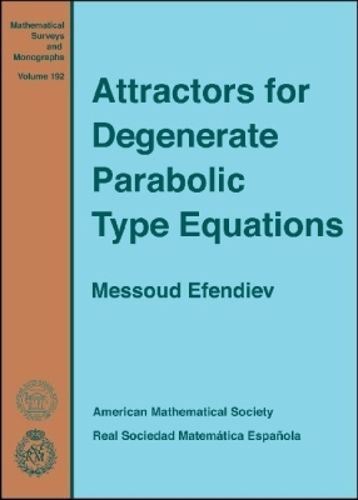•# Attractors for Degenerate Parabolic Type Equations - Mathematical Surveys and Monographs (Hardback)

(author)
£97.95
Hardback 225 Pages / Published: 30/10/2013
• We can order this

Usually dispatched within 2 weeks

This book deals with the long-time behaviour of solutions of degenerate parabolic dissipative equations arising in the study of biological, ecological, and physical problems. Examples include porous media equations, \$p\$-Laplacian and doubly nonlinear equations, as well as degenerate diffusion equations with chemotaxis and ODE-PDE coupling systems. For the first time, the long-time dynamics of various classes of degenerate parabolic equations, both semilinear and quasilinear, are systematically studied in terms of their global and exponential attractors. The long-time behaviour of many dissipative systems generated by evolution equations of mathematical physics can be described in terms of global attractors. In the case of dissipative PDEs in bounded domains, this attractor usually has finite Hausdorff and fractal dimension. Hence, if the global attractor exists, its defining property guarantees that the dynamical system reduced to the attractor contains all of the nontrivial dynamics of the original system. Moreover, the reduced phase space is really ``thinner'' than the initial phase space. However, in contrast to nondegenerate parabolic type equations, for a quite large class of degenerate parabolic type equations, their global attractors can have infinite fractal dimension.

The main goal of the present book is to give a detailed and systematic study of the well-posedness and the dynamics of the semigroup associated to important degenerate parabolic equations in terms of their global and exponential attractors. Fundamental topics include existence of attractors, convergence of the dynamics and the rate of convergence, as well as the determination of the fractal dimension and the Kolmogorov entropy of corresponding attractors. The analysis and results in this book show that there are new effects related to the attractor of such degenerate equations that cannot be observed in the case of nondegenerate equations in bounded domains. This book is published in cooperation with Real Sociedad Matematica Espanola (RSME).

Publisher: American Mathematical Society
ISBN: 9781470409852
Number of pages: 225